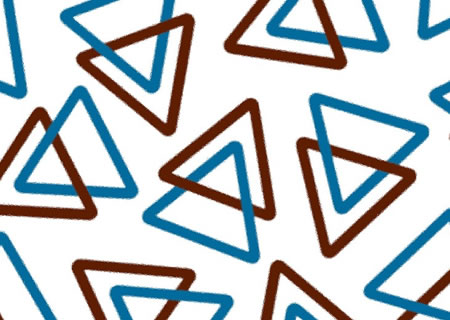Home Free Lessons Magic Triangles• Author:
• Posted:
• Topics:
, ,
• Type:
• Keywords:

#### Overview

Materials: Pencil and paper
Common Core: K.OA.A.2, K.OA.A.4, 1.OA.A.2, 1.OA.B.3, 1.OA.B.4, 1.OA.C.5,1.OA.C.6, 1.OA.D.8, 2.OA.B.2, MP1.

Magic Triangles are a simple addition/missing addend problem that creates opportunities for higher level thinking. Kids must place the numbers 1 through 6 on the outside of a triangle to make the numbers on each side sum to the same amount.

#### Why We Love Magic Triangles

Magic Triangles are really a series of problems that grow gradually harder. These are a perfect way to practice one-digit addition in a context that promotes logical thinking and problem-solving.

#### The Launch

The teacher presents the first problem:
is it possible to place each number 1 through 6 around the outside of a triangle and make the numbers on each side add up to 10? With the students help, the teacher tries the problem out.

Teacher: Let’s see… I could put the 6 here, and the 3 here.
Then what would need to go in this spot?
[calls on a student]
Student: It would have to be a 1, since 6 + 3 = 9, and that’s 1 away from 10.
Teacher: So now I still have a 2, 4 and 5 to place. Where should I put them?
Student: Put the 4 on the top. Then 4 + 6 = 10.
Teacher: All right. Then what goes in the circle on the right?
I’ve got a 4 and a 1 on that side already.
What plus four plus one equals ten?
Think about it, then share with your neighbor what you think the answer is, and explain why you think that’s right. [Kids pair andshare.]

Student: It has to be a 5, since 4 + 1 = 5, and 5 plus 5 is 10.
Teacher: Then what goes in the last spot?
Student: Well… 2. But that can’t be right!
Teacher: Hmmm. You’re right. 6 + 2 + 4 = 12, so we made a mistake on that side.
We need to move something around.
Maybe putting the 6 and 3 where I did to start was a mistake.
What else could I have tried?
Student: Maybe you should have moved the 3 to the top.
Student: You could have put the 1 in the corner to start.
Teacher: Maybe some of those will work. Maybe they won’t.
We won’t know until we try them out.
I’m going to give you the chance to do that now.
You can take a worksheet or draw your own, and see if you can solve the Magic Triangle puzzle.
It is possible! Once you get an answer, there are some even trickier ones to try out. Let’s go!

#### The Wrap

There are many extensions of Magic Triangles—see the attached worksheets—but after you’ve given the students some time (20-30 minutes or so), you can expect that almost everyone will have finished the initial problem, and tried out some of the others. Bring the students together and ask for solutions. Some followups to consider during the discussion:

• How many solutions are there? Is there some connection between the solutions?
• Does it matter what numbers go in the corners?
• What strategies did students use to solve the Magic Triangle?
• What are some ways we could extend this problem?

(i.e., longer sides to the triangle, magic squares, pentagons, etc.)

#### Tips for the Classroom

1. Make sure you demonstrate filling in a Magic Triangle wrong first in the demonstration.
This will be far more motivating to the students than doing one right.
2. To differentiate down for students who need more concrete help, use blocks, tiles, or cubes to count out piles of each size.
You can also let students drop the restriction that all the numbers must be different, and just let them try to make every side add up to ten.